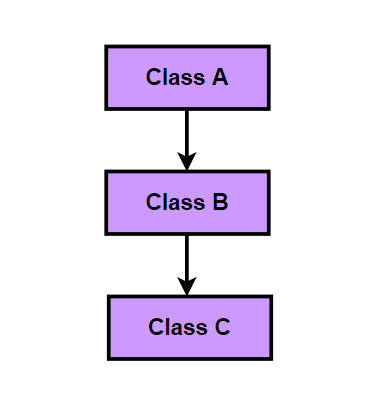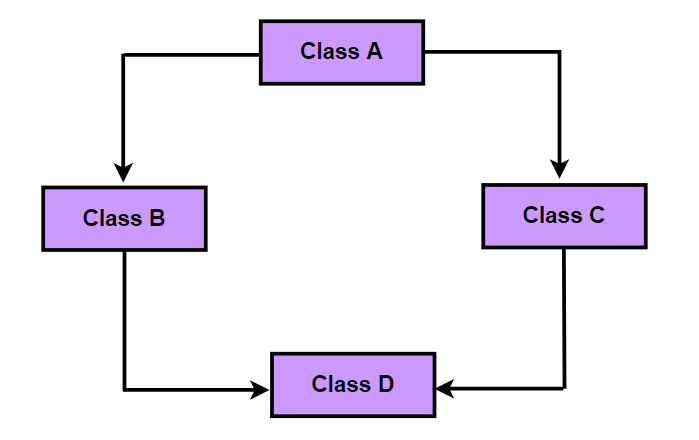# Java Inheritance

In this tutorial, we will learn about inheritance in Java with the help of examples.

### Inheritance

Java Inheritance is one of the key feature of OOP (Object Oriented Programming). Inheritance can be defined as the process where one class is allowed to inherit the features (fields and methods) of another class.

Inheritance supports the concept of “reusability”, i.e. The code that is present in the parent class doesn’t need to be written again in the child class.

The class which inherits the features of other class is known as sub class (or a derived class or a child class).

The class whose features are inherited is known as super class ( or a base class or  a parent class).

##### Syntax
```class Subclass-name extends Superclass-name
{
//methods and fields
}```

In Java, we use the extends keyword to inherit features from a super class in sub class.

##### Example
```//Calculator is a super class
class Calculator {

//Calculator class members
public int add(int x, int y) {
return x + y;
}

public int sub(int x, int y) {
return x - y;
}
}

public int sqr(int x) {
return x * x;
}

public double sqrt(float y) {
return Math.sqrt(y);
}

}

public class Main {

public static void main(String args[]) {

System.out.println("The difference between the given numbers: " + calcAdv.sub(30, 20));
System.out.println("The square of a given number: " + calcAdv.sqr(10));
System.out.println("The square root of a given number: " + calcAdv.sqrt(25));

}
}```
##### Output
```The sum of the given numbers: 30
The difference between the given numbers: 10
The square of a given number: 100
The square root of a given number: 5.0```
##### Example explained
• Here, we have inherited a subclass CalcAdv from super class Calculator. The CalcAdv class inherits the methods add() and sub() from the Calculator class.
• When an object of CalcAdv class is created, a copy of all methods and fields of the super class acquire memory in this object. That is why by using the object of the subclass we can also access the members of a super class.
• Hence, objects of the CalcAdv class can access the members of Calculator class.

### Types of Inheritance in Java

There are various types of inheritance in Java:

#### Single Inheritance

In single Inheritance, a class inherits features of another class (one class only).In above diagram, Class B extends only Class A. Class A is a super class and Class B is a Sub class.

##### Example: Single Inheritance
```//Person is a super class
class Person {

private String name;
private int age;

public String getName() {
return name;
}

public void setName(String name) {
this.name = name;
}

public int getAge() {
return age;
}

public void setAge(int age) {
this.age = age;
}

}

//Student is a sub class
class Student extends Person {

private int rollno;

public int getRollno() {
return rollno;
}

public void setRollno(int rollno) {
this.rollno = rollno;
}

}

public class Main {
public static void main(String[] args) {
//creating Student instance
Student student = new Student();
student.setRollno(101);
student.setName("Arun Verma");
student.setAge(18);

System.out.println("Rollno: " + student.getRollno());
System.out.println("Name: " + student.getName());
System.out.println("Age: " + student.getAge());

}
}```
##### Output
```Rollno: 101
Name: Arun Verma
Age: 18```

#### Multilevel Inheritance

In Multilevel Inheritance, a sub class inherits all the properties and behaviours of more than one super class at multiple levels.In above diagram, Class A is a super class of Class B and Class B is a super class of Class C or Class C is a subclass of Class B and Class B is a subclass of Class A.

##### Example: Multilevel Inheritance
```//Person is a super class of Student
class Person {
private String name;
private int age;

public String getName() {
return name;
}

public void setName(String name) {
this.name = name;
}

public int getAge() {
return age;
}

public void setAge(int age) {
this.age = age;
}

}
//Student is a sub class of Person and super class of School
class Student extends Person {

private int rollno;

public int getRollno() {
return rollno;
}

public void setRollno(int rollno) {
this.rollno = rollno;
}

}
//School is a sub class of Student
class School extends Student {

private String schoolName;
private String standard;
private char section;

public String getSchoolName() {
return schoolName;
}

public void setSchoolName(String schoolName) {
this.schoolName = schoolName;
}
}

public class Main {

public static void main(String[] args) {
//creating School object
School school = new School();
school.setName("Rohit Verma");
school.setRollno(102);
school.setSchoolName("Global Public School");

System.out.println("Name: " + school.getName());
System.out.println("Rollno: " + school.getRollno());
System.out.println("School Name: " + school.getSchoolName());

}
}```
##### Output
```Name: Rohit Verma
Rollno: 102
School Name: Global Public School```

#### Hierarchical Inheritance

In hierarchical inheritance, two or more classes inherits a single class.In above diagram, class B, C, D inherit the same class A.

##### Example: Hierarchical Inheritance
```//Person is a super class of Student and Teacher
class Person {
private String name;
private int age;

public String getName() {
return name;
}

public void setName(String name) {
this.name = name;
}

public int getAge() {
return age;
}

public void setAge(int age) {
this.age = age;
}

}
//Student is a sub class of Person
class Student extends Person {

private int rollno;

public int getRollno() {
return rollno;
}

public void setRollno(int rollno) {
this.rollno = rollno;
}

}
//Teacher is a sub class of Person
class Teacher extends Person {

private int salary;

public int getSalary() {
return salary;
}

public void setSalary(int salary) {
this.salary = salary;
}
}

public class Main {
public static void main(String[] args) {
//creating Student object
Student student = new Student();
student.setRollno(101);
student.setName("Arun Verma");
student.setAge(18);
//creating Teacher object
Teacher teacher = new Teacher();
teacher.setSalary(15000);
teacher.setName("Sachin Sharma");
teacher.setAge(30);

System.out.println("Student Name: " + student.getName() + " | " + "Rollno: " + student.getRollno() + " | " + "Age: " + student.getAge());
System.out.println("Teacher Name: " + teacher.getName() + " | " + "Salary: " + teacher.getSalary() + " | " + "Age: " + teacher.getAge());

}
}```
##### Output
```Student Name: Arun Verma | Rollno: 101 | Age: 18
Teacher Name: Sachin Sharma | Salary: 15000 | Age: 30```

#### Multiple Inheritance

In Multiple inheritance, one class can have more than one super class and inherit features from all parent classes.In above diagram, Class C extends Class A and Class B both.

#### Hybrid Inheritance

Hybrid Inheritance is a mix of two or more of the above types of inheritance.Note: Java does not support Multiple and Hybrid inheritance with classes. In java, we can achieve both inheritance only through Interfaces.